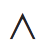## 代码高亮

import os
import pdb
from collections import OrderedDict
import numpy as np

def safe_mkdir(path):
"""make dir safely"""
if not os.path.exists(path):
os.makedirs(path)


Jecklly原生的代码高亮功能比较少，比如无法高亮某一特定行，而且带行号的布局也不好看。 修改后使用基于javascript的通用代码高亮插件syntaxhighlighter。 插入的代码和效果如下

<pre class="brush: python highlight:'[3,5]'">
import os
import pdb
from collections import OrderedDict
import numpy as np

def safe_mkdir(path):
"""make dir safely"""
if not os.path.exists(path):
os.makedirs(path)
</pre>

import os
import pdb
from collections import OrderedDict
import numpy as np

def safe_mkdir(path):
"""make dir safely"""
if not os.path.exists(path):
os.makedirs(path)


# 下面的{\% 和 %\} 均应没有"\", 这里如果写作没有"\"的样子则会执行这段代码...
{\% assign thisCount= thisCount|plus:1 %\}
{\% include new_highlight.html language="python" title="longCode.py" language_show="python"
first-line="1" html-script="false"
smart-tab="true" tabSize="4" count=thisCount %\}
# this is a long long long code, you can ^^^^^^ try to fold it
import time
import re
import inspect
import os

class logFileClass():
def __init__(self, filePath):
self.newLogFlag=1
self.filePath = filePath
....omit below.....
</pre> #用</pre>来结束代码高亮


longCode.pypython


# this is a long long long code, you can ^^^^^^ try to fold it
import time
import re
import inspect
import os

class logFileClass():
def __init__(self, filePath):
self.newLogFlag=1
self.filePath = filePath
logFileDir = os.path.dirname(filePath)
if logFileDir not in ['', '.'] and not os.path.exists(logFileDir):
os.mkdir(logFileDir)
if self.newLogFlag==1:
f = open(self.filePath,'a')
f.write('============================='+time.strftime('%Y-%m-%d %H:%M:%S')+'====================================\n')
f.close()
self.newLogFlag=0
def log(self, text):
with open(self.filePath,'a') as f:
if text[-1] != '\n':
text = text+'\n'
text = time.strftime('%Y-%m-%d %H:%M:%S ')+text
f.write(text)
def print(self, text):
print(text)
with open(self.filePath,'a') as f:
if text[-1] != '\n':
text = text+'\n'
text = time.strftime('%Y-%m-%d %H:%M:%S ')+text
f.write(text)

class logFunctionCallClass():
def __init__(self, logFile=None):
self.n=1;
self.initDepth = len(inspect.stack())
if logFile:
self.logFile = logFile

def logit(self,path=None):
if not self.logFile:
if not path:
raise "no path for log file"
else:
if not path:
path=self.logFile
logFileDir = os.path.dirname(path)
if logFileDir and not os.path.exists(logFileDir):
os.mkdir(os.path.dirname(logFileDir))
if self.n==1:
f = open(path,'a')
f.write('============================='+time.strftime('%Y-%m-%d %H:%M:%S')+'====================================\n')
f.close()
self.n=0

def maxLength(inStr,maxLength=50):
N = len(inStr)
if N>maxLength:
return inStr[:maxLength-6] + '......'
else:
return inStr

def toPrint(u):
us = re.findall(r'\\\w(\w{4})',unicode(u))
uus = [unichr(int('0x'+each,16)) for each in us]
uuus = u
for i in uus:
uuus = re.sub(r'\\(\w{5})',i,uuus,count=1)
return uuus

def _logit(func):
def __logit(*args, **kwargs):
depth = len(inspect.stack())-self.initDepth
p0 = time.strftime('%H:%M:%S')
p1 = func.func_name
p2 = '('
for i in args:
if type(i)==str:
p2 = p2 + '"' + maxLength(i.decode('utf8')) + '"' + ', '
elif type(i)==unicode:
p2 = p2 + '"' + maxLength(i) + '"' + ', '
else:
p2 = p2 + maxLength(unicode(i)) + ', '
try:
p2 = re.findall(r'<.*>,(.*)',p2)
p2 = '('+p2
except:
pass
p3 = ' '
for i in kwargs:
if type(kwargs[i])==str:
p3 = p3 + i + ' = ' + '"' + maxLength(kwargs[i].decode('utf8')) + '"' + ', '
elif type(kwargs[i])==unicode:
p3 = p3 + i + ' = ' + '"' + maxLength(kwargs[i]) + '"' + ', '
else:
p3 = p3 + i + ' = ' + maxLength(unicode(kwargs[i])) + ', '

pn = depth * "  " + p0 + '  ' + p1 + p2 + p3[0:-2] + ')\n'
pn = toPrint(pn)
ret = func(*args, **kwargs)
#print pn
f = open(path,'a')
f.write(pn.encode('utf8'))
f.close()
return ret
return __logit
return _logit


## 博文分类

• 含有较多原创成分、需要较长创作周期的博文: 之前的博文大多都是这样的。这类博文放在博客首页上，可能会成为有生之年系列。

• 技术类博文: 现在已经逐渐习惯用Markdown语法记录工作中的技术细节。这些东西可以很容易的转化为博文，故此类博文可能更新频率会高一些，而且可以保证都是干货。这些博文放在轮子目录中。

• 碎碎念: 我初高中的时候，网易博客、QQ空间的使用人数开始多起来，wordpress博客也刚开始在中国流行起来。我现在还能记得自己熬夜(12:00睡觉…)写完第一篇网易博文后的兴奋之情，那个时候博客都是当日记写的…..后来社交网络兴起，碎片化文字时代来临，人们热衷于向别人高频率的展示自己的生活，到处刷着存在感，却很少真正的为自己记录着些什么。 宜居带目录中将会是我的生活随笔，我为它添加了简单的图灵测试，仅仅是不想让搜索引擎收录。这部分的更新主要看心情。

To be continue…